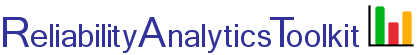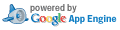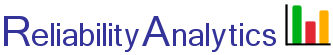# Weibull Analysis

Weibull analysis (life data analysis) allows for making predictions about the life expectancy of a product. The technique is valuable because predictions can be made based on a relatively small sample of test or field data. The data sample is fitted to a Weibull distribution using "Weibull analysis." Once the data is fitted to a Weibull distribution, the probability of survival can be estimated for any point in time. This calculator performs a basic Weibull analysis. The analysis results can be used to answer questions such as:

1. When will my product begin to fail (e.g., L10% life)?
2. What is a reasonable time for a product warranty?
3. Do my products exhibit premature failure (e.g., infant mortality)?
4. Do my products fail randomly over time, or are the failure characteristics more wear-out related?
5. At what point will most products fail?

Tip: For a quick demonstration, select a test data set from the last pull-down in the Options area (#2) and click calculate.

The data input format (time-to-failure, box 1 below) is a failure time followed by either an "f" or an "s", indicating a failure or suspension (i.e., item did not fail), one record per line. Box #1 is prefilled with example input data for eight test items. The first item failed at 20 hours, the second was taken off test (suspended) at 42 hours and the final item failed at 139 hours.

The following provides an example for grouped, or interval data input. The columns are "time-to-failure", "f" or "s" indicating a failure or suspension, "g" indicating grouped data, followed by the number of items in the group. The following shows example input for 93 items placed on test. Between test start and 10 hours, one item failed. Between 10 and 20 hours 11 failures occurred. After 50 hours 8 items still did not fail and the test was stopped, indicated by an "s" in the second column of the final input line. The analysis results from this input assume that failures occur at the end of the interval. If failures can occur anytime during the interval, then a more accurate approach is to enter mid-point times for the interval (i.e., change the first four times to 5, 15, 25 and 35).

10 f g 1
20 f g 11
30 f g 28
40 f g 45
50 s g 8

Data entered into box #1 below can be separated by spaces, tabs (e.g., copy paste from Microsoft Excel), or no space at all (e.g., 10fg1). Because the Weibull plot starts at a minimum time value of one, if fractional time values less than 1.0 are being analyzed, such as a failure at 0.5 hours, all time values should first be scaled upward by converting to minutes (i.e., multiply all times by 60 minutes/hour).

Example Excel template
Example Excel template, grouped data

Excel Warranty Data Analysis Template.xls

## Calculation Inputs:

1. Time-to-failure input, one record per line.

Input is limited to approximately 1,600 lines for Weibull parameter analysis. Weibull probability plot is limited to approximately 300 points.
Remove commas from input data.

2. Options:

Decimal places
Confidence level:
Summary table *
Increment/order table
Box 1 input on output page

Plots: Weibull, F(t), R(t), f(t), h(t), Failure timeline
Parameters used for plots: RRY MLE RRX
Rank Regression on Y (RRY), Rank Regression on X (RRX), Maximum Liklihood Estimate (MLE)
Salient points

Time:
Shows dot on plots. Enter value between min and max life, or an encoded number, such as F10 for 10% life.

X-axis label:

Use a test data set:

Selecting a test data set will overide all other inputs above.

* If a browser page error occurs with large data sets, try unchecking the summary table option.

## Featured Reference:The New Weibull Handbook: Reliability and Statistical Analysis for Predicting Life, Safety, Supportability, Risk, Cost and Warranty Claims

Toolkit Homereliabilityanalytics.com

### References:

1. MIL-HDBK-338, Electronic Reliability Design Handbook.
2. Nelson, Wayne, Applied Life Data Analysis
3. Dodson, Bryan, The Weibull Analysis Handbook.
4. Abernethy, Robert, The Weibull Analysis Handbook.
5. Bazovsky, Igor, Reliability Theory and Practice.
6. O'Connor, Patrick, D. T., Practical Reliability Engineering.
7. http://en.wikipedia.org/wiki/Weibull_distribution
8. http://en.wikipedia.org/wiki/Maximum_likelihood
9. Weibull Distribution, NIST Engineering Statistics Handbook .
10. Khan Academy, Probability density functions for continuous random variables..
11. Barringer, Paul, Barringer & Associates, Inc., database of typical Weibull shape and characteristic life parameters (wdbase), Feb. 22, 2010 (no longer available online).
12. http://reliawiki.org/index.php/Weibull_Distribution_Examples
13. Kececioglu, Dimitri, Reliability & Life Testing Handbook.
14. NIST, Confidence Intervals, http://www.itl.nist.gov/div898/handbook/prc/section2/prc241.htm.
15. Confidence Intervals, Electronic Reliability Design Handbook.
16. Bloch, Heinz P. Introduction to Machinery Reliability Assessment.
17. Jardine, Andrew K.S.; Tsang, Albert H.C.Maintenance, Replacement, and Reliability: Theory and Applications.